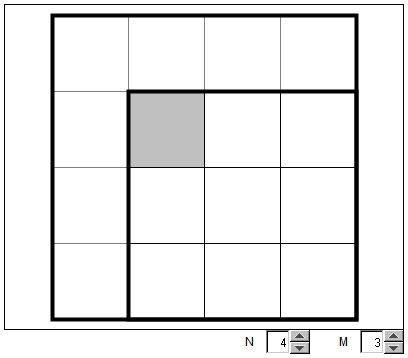# Counting Squares in a Square

How many grid squares are there in a grid square of size $N\times N$?

Obviously, there is the $N\times N$ square itself and $N^2$ small, $1\times 1$ squares. But, for $N,$ say equal to $5,$ there are also $2\times 2,$ $3\times 3,$ and $4\times 4$ squares. In general, for the $N\times N$ square there are smaller squares of all possible sizes, starting with $1\times 1$ all through $N\times N$. How do you count those?

The applet bellow suggests a way of counting $M\times M$ squares contained in an $N\times N$ square, $N \ge M.$ In the applet, the $M\times M$ square is draggable. Try it and see what happens. Imagine the $1\times 1$ square in the upper left corner of the moveable square is filled with paint which leaks when the square moves, but only if it remains within the borders of the $N\times N$ square.

### If you are reading this, your browser is not set to run Java applets. Try IE11 or Safari and declare the site https://www.cut-the-knot.org as trusted in the Java setup.What if applet does not run?The position of an $M\times M$ square is fully determined by its upper left $1\times 1$ piece. (The upper left corner is chosen for convenience. Any other small square inside a bigger one determines uniquely the position of the latter.) Inside the $N\times N$ square this $1\times 1$ piece may occupy a limited number of positions. These form a square of side $N - M + 1$ in the upper right corner of the $N\times N$ square. It follows that the number of $M\times M$ squares inside the $N\times N$ square equals $(N - M + 1)\times (N - M + 1).$

### If you are reading this, your browser is not set to run Java applets. Try IE11 or Safari and declare the site https://www.cut-the-knot.org as trusted in the Java setup.What if applet does not run?

The total number $T$ of grid squares within the $N\times N$ grid is the sum:

\begin{align}\displaystyle T &= \sum_{n=1}^{N}(N-n+1)^2 \\ &= \sum_{n=1}^{N}N^2, \end{align}

the sum of the first $N$ squares. This we know to be

$\displaystyle T=\frac{N(N + 1)(2N + 1)}{6}.$